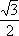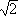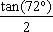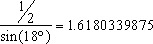## Finding Face-to-Face Angles

Finding face to face angles is important to determining which shapes tile and which do not. Our strategy for finding face to face angles is as follows. The face to face angle is the angle between faces, along a plane that is perpendicular to both faces. This cutting plane is also perpendicular to the common edge that the faces share. The next step was to find lines perpendicular to the common edge, each one along one of the two faces. These two lines could be connected into a triangle. Once the lengths of the lines was found, it was easy to use trigonometry to find the angles of the triangle, also the face-to-face angle. For ease, assume that the side length of the polyhedrons in question is 1. The face-to-face angle on a cube is 90°, because the cubes faces are all perpendicular to each other, and thus by definition the face-to-face angle must be 90°.

### Face-to-Face angles on the tetrahedron.

The triangle that gives us the face-to-face angle has 2 sides with length(the altitude of the faces), and the third side, opposite the face-to-face angle, is 1. This yields an angle of approximately 70.5.

### Face-to-Face angles on the octahedron.

The triangle that gives us the face-to-face angle in the octahedron has two sides with length(again the altitude of the faces). Since the vertexes of the octahedron can be seen as touching the centers of the faces of the cube, the distance between two opposite points can be thought of as the height of the cube with diagonal of 2:. The angle between faces of the octahedron is approximately 109.5.

### Face-to-face angles on the icosahedron.

The angle between faces on an icosahedron can be found in the following way. Take the 5 sided pyramid formed by 5 of the triangular faces and remove it- the rest of the shape wont be necesary for finding the face-to-face angle. Label the five vertices of the pentagonal base of the pyramid A,B,C,D, and E. Label the tip of the pyramid X. The two sides between which the angle will be found are ABX and AXE. The midpoint of AX is F. So the triangle containing the face-to-face angle is BFE. Since the lines BF and FE are altitudes of the faces ABX and AXE, they have length. The line BE can be found with trigonometry- it is equal to 2·sin(54°) or 1.6180339875. 3 Now that we know the three sides of BFE, we can use trigonometry again to calculate the angle at point F. The angle is approximately 138.189685104.

### Face-to-face angles on the dodecahedron.

The angle between faces on an dodecahedron can be found in the following way. Label two adjacent faces ABCDE and HAEFG so that they meet along the line AE. Bisect AE at point I. GI and IC are two lines along the triangles containing the face-to-face angle. The altitude of a pentagon with side length 1 isor 1.53884176859.

The third side of the triangle GIC is a line in the pentagon formed by G,E,C and two other points on the dodecahedron. The side lengths in this pentagon are diagonals of the faces. Their side length is(Once again, the golden mean). A diagonal in this pentagon, congruent to a pentagon with side length 1 magnified by 1.6180339875, is 1.61803398752. Curiously, this number is 2.6180339875 (See! Something wacky going on). Using trigonometry again, the face-to-face angle of the triangles with side lengths 1.53884176859 and 2.6180339875 is 116.565051178.### What do we do with these numbers?

Since the face-to-face angles on a cube are rational, and divide 360 into an integer value, cubes can tile alone. The face-to-face angles on a octahedron and a tetrahedron are both irrational, but two of each add up to 360 evenly. Since the face-to-face angles on icosahedrons and dodecahedrons do not add up to 360 evenly, they cannot tile with theselves or with each other. Since they do not add up with any other shapes, they cannot tile space at all. And since all truncations of the icosahedron and the dodecahedron contain pentagons, they could only tile with themselves. It remains to be seen if there is a tiling of space with some of the members of the dodecahedron-icosahedron family.